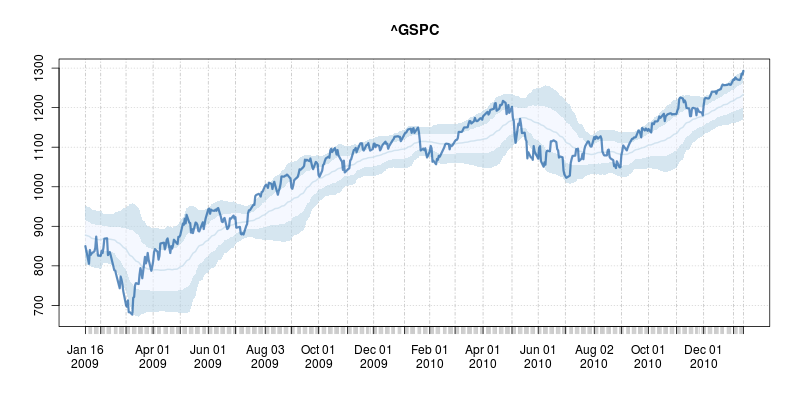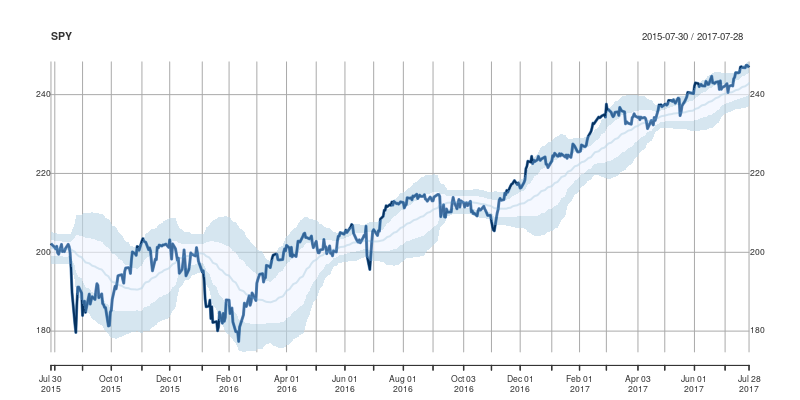### Updated overbought/oversold plot function

A good six years ago I blogged about `plotOBOS()` which charts a moving average (from one of several available variants) along with shaded standard deviation bands. That post has a bit more background on the why/how and motivation, but as a teaser here is the resulting chart of the SP500 index (with ticker ^GSCP):The code uses a few standard finance packages for R (with most of them maintained by Joshua Ulrich given that Jeff Ryan, who co-wrote chunks of these, is effectively retired from public life). Among these, xts had a recent release reflecting changes which occurred during the four (!!) years since the previous release, and covering at least two GSoC projects. With that came subtle API changes: something we all generally try to avoid but which is at times the only way forward. In this case, the shading code I used (via `polygon()` from base R) no longer cooperated with the beefed-up functionality of `plot.xts()`. Luckily, Ross Bennett incorporated that same functionality into a new function `addPolygon` --- which even credits this same post of mine.

With that, the updated code becomes

``````## plotOBOS -- displaying overbough/oversold as eg in Bespoke's plots
##
## Copyright (C) 2010 - 2017  Dirk Eddelbuettel
##
## This is free software: you can redistribute it and/or modify it
## the Free Software Foundation, either version 2 of the License, or
## (at your option) any later version.

suppressMessages(library(quantmod))     # for getSymbols(), brings in xts too
suppressMessages(library(TTR))          # for various moving averages

plotOBOS <- function(symbol, n=50, type=c("sma", "ema", "zlema"),
years=1, blue=TRUE, current=TRUE, title=symbol,
ticks=TRUE, axes=TRUE) {

today <- Sys.Date()
if (class(symbol) == "character") {
X <- getSymbols(symbol, from=format(today-365*years-2*n), auto.assign=FALSE)
x <- X[,6]                          # use Adjusted
} else if (inherits(symbol, "zoo")) {
x <- X <- as.xts(symbol)
current <- FALSE                # don't expand the supplied data
}

n <- min(nrow(x)/3, 50)             # as we may not have 50 days

sub <- ""
if (current) {
xx <- getQuote(symbol)
x <- rbind(x, xt)
sub <- paste("Last price: ", xx\$Last, " at ",
}

type <- match.arg(type)
xd <- switch(type,                  # compute xd as the central location via selected MA smoother
sma = SMA(x,n),
ema = EMA(x,n),
zlema = ZLEMA(x,n))
xv <- runSD(x, n)                   # compute xv as the rolling volatility

strt <- paste(format(today-365*years), "::", sep="")
x  <- x[strt]                       # subset plotting range using xts' nice functionality
xd <- xd[strt]
xv <- xv[strt]

xyd <- xy.coords(.index(xd),xd[,1]) # xy coordinates for direct plot commands
xyv <- xy.coords(.index(xv),xv[,1])

n <- length(xyd\$x)
xx <- xyd\$x[c(1,1:n,n:1)]           # for polygon(): from first point to last and back

if (blue) {
blues5 <- c("#EFF3FF", "#BDD7E7", "#6BAED6", "#3182BD", "#08519C") # cf brewer.pal(5, "Blues")
fairlylight <<- rgb(189/255, 215/255, 231/255, alpha=0.625) # aka blues5
verylight <<- rgb(239/255, 243/255, 255/255, alpha=0.625) # aka blues5
dark <<- rgb(8/255, 81/255, 156/255, alpha=0.625) # aka blues5
## buglet in xts 0.10-0 requires the <<- here
} else {
fairlylight <<- rgb(204/255, 204/255, 204/255, alpha=0.5)  # two suitable grays, alpha-blending at 50%
verylight <<- rgb(242/255, 242/255, 242/255, alpha=0.5)
dark <<- 'black'
}

plot(x, ylim=range(range(x, xd+2*xv, xd-2*xv, na.rm=TRUE)), main=title, sub=sub,
major.ticks=ticks, minor.ticks=ticks, axes=axes) # basic xts plot setup
addPolygon(xts(cbind(xyd\$y+xyv\$y, xyd\$y+2*xyv\$y), order.by=index(x)), on=1, col=fairlylight)  # upper
addPolygon(xts(cbind(xyd\$y-xyv\$y, xyd\$y+1*xyv\$y), order.by=index(x)), on=1, col=verylight)    # center
addPolygon(xts(cbind(xyd\$y-xyv\$y, xyd\$y-2*xyv\$y), order.by=index(x)), on=1, col=fairlylight)  # lower
lines(xd, lwd=2, col=fairlylight)   # central smooted location
lines(x, lwd=3, col=dark)           # actual price, thicker
}``````

and the main change are the three calls to `addPolygon`. To illustrate, we call `plotOBOS("SPY", years=2)` with an updated plot of the ETF representing the SP500 over the last two years: